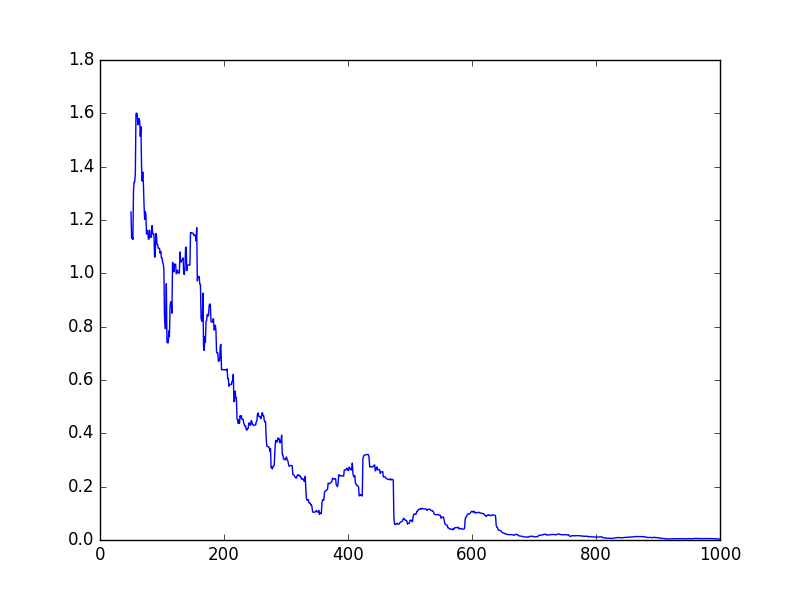# Training and Convergence

A key component of most artificial intelligence and machine learning is looping, i.e. the system improving over many iterations of training. A very simple method to train in this way is just to perform updates in a for loop. We saw an example of this way back in lesson 2:

We can alter this workflow to instead use a variable as the convergence loop, such as in the following:

The major change here is that the loop is now a `while` loop, continuing to loop while the test (using `tf.less` for a less-than-test) is true. Here, we test if `x` is less than a given threshold (stored in a constant), and if so, we continue looping.

Any machine learning library must have a gradient descent algorithm. I think it is a law. Regardless, Tensorflow has a few variations on the theme, and they are quite straight forward to use.

Gradient Descent is a learning algorithm that attempts to minimise some error. Which error do you ask? Well that is up to us, although there are a few commonly used methods.

The major line of interest here is `train_op = tf.train.GradientDescentOptimizer(0.01).minimize(error)` where the training step is defined. It aims to minimise the value of the `error`Variable, which is defined earlier as the square of the differences (a common error function). The `0.01` is the step it takes to try learn a better value.

An important note here is that we are optimising just a single value, but that value can be an array. This is why we used `w` as the Variable, and not two separate Variables `a` and `b`.

# Other Optimisation

TensorFlow has a whole set of types of optimisation, and has the ability for your to define your own as well (if you are into that sort of thing). For the API of our how to use them, see this page. The listed ones are:

• MomentumOptimizer
• FtrlOptimizer
• RMSPropOptimizer

Other optimisation methods are likely to appear in future releases of TensorFlow, or in third-party code. That said, the above optimisations are going to be sufficient for most deep learning techniques. If you aren’t sure which one to use, use GradientDescentOptimizer unless that is failing.

# Plotting the error

We can plot the errors after each iteration to get the following output:The code for this is a small change to the above. First, we create a list to store the errors in. Then, inside the loop, we explicitly compute both the `train_op` and the `error`. We do this in a single line, so that the error is computed only once. If we did this is separate lines, it would compute the error, and then the training step, and in doing that it would need to recompute the error.

Below I’ve put the code just for below the `tf.global_variables_initializer()` line from the previous program – everything above this line is the same.

You may have noticed that I take a windowed average here – using `np.mean(errors[i-50:i])` instead of just using `errors[i]`. The reason for this is that we are only doing a single test inside the loop, so while the error tends to decrease, it bounces around quite a bit. Taking this windowed average smooths this out a bit, but as you can see above, it still jumps around.

1) Create a convergence function for the k-means example from Lesson 6, which stops the training if the distance between the old centroids and the new centroids is less than a given epsilon value.

2) Try separate the `a` and `b` values from the Gradient Descent example (where `w` is used).

3) Our example trains on just a single example at a time, which is inefficient. Extend it to learn using a number (say, 50) of training samples at a time.